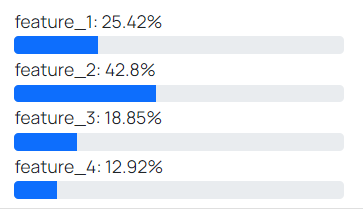# Model Info

After creating the model, you can check the model information out. There are lots of statistical parameters here. You may find it difficult to understand the explanations of every single parameter. Here are some of the main parameters you should look at.

Learn: Calculated value of the metric for the training dataset.

Test: Calculated value of the metric for the validation dataset.

For example, if the eval metric is RMSE when your output is numerical then these metric shows the RMSE which means "root-mean-square error", a frequently used measure of the differences between values predicted by a model or an estimator and the values observed.

If your model output is categorical, then this metric measures the performance of a classification model whose output is a probability value between 0 and 1. Log-loss is indicative of how close the prediction probability is to the corresponding actual/true value. The more the predicted probability diverges from the actual value, the higher is the log-loss value.

Learning time: The time it takes to complete the learning.

Feature names: Titles of the data columns which used in the model.

Feature importance: This list shows how much percentage on average the output prediction changes if the feature data changes.Model size: The size of the model file in MB.

Data size: The size of the data file in MB.

Algorithm: The ML algorithm used in the model.

Tree Count: The number of trees used in the model. If you set the "use best model" on, this value will be defined automatically and may be less than the iteration count.

eval_metric: Evaluation metric of the model.

iterations: The maximum tree number that can be created when learning process. May be greater than tree count if best model set is on.

class_names: The output values of the prediction model.

depth: It is depth of the tree using in the model.

learnin_rate: It is for reducing the gradient step and affects the overall time of training. It can be in the range of 0 and 1.

Next: API